Courses

# Displacement, Velocity & Acceleration Analysis of Plane Mechanisms Notes | EduRev

## Mechanical Engineering : Displacement, Velocity & Acceleration Analysis of Plane Mechanisms Notes | EduRev

The document Displacement, Velocity & Acceleration Analysis of Plane Mechanisms Notes | EduRev is a part of the Mechanical Engineering Course Theory of Machines (TOM).
All you need of Mechanical Engineering at this link: Mechanical Engineering

Introduction

The study of velocity analysis involves the linear velocities of various points on different links of a mechanism as well as the angular velocities of the links.

• The velocity analysis is the prerequisite for acceleration analysis which further leads to force analysis of various links of a mechanism.
• To facilitate such study, a machine or a mechanism is represented by a skeleton or a line diagram, commonly known as a configuration diagram.
• Velocities and accelerations in machines can be determined either analytically or graphically.

Absolute and Relative Motions

• All motions are relative since an arbitrary set of axes or planes is required to define a motion.
• Usually, the earth is taken to be a fixed reference plane and all motions relative to it are termed absolute motions.

Motion of A Link (Relative Velocity Method)

Let a rigid link OA, of length r, rotate about a fixed point O with a uniform angular velocity ω rad/s in the counter-clockwise direction. OA turns through a small angle δθ in a small interval of time δt. Then A will travel along the arc AA’ as shown in Fig.1 (b) below.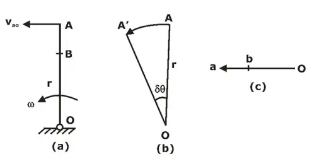Fig. 1: Motion of a Link

Velocity of A relative to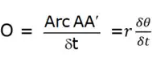In the limits, when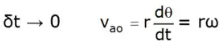The direction of Vao is along the displacement of A. Also, as δt approaches zero (δt → 0), AA’ will be perpendicular to OA. Thus, velocity of A is ωr and is perpendicular to OA. This can be represented by a vector oa (Fig.1c).
Consider a point B on the link OA.
Velocity of B = ω. OB perpendicular to OB.
If ob represents the velocity of B, it can be observed that
i.e., b divides the velocity vector in the same ratio as B divides the link.
Remember, the velocity vector Vao [Fig. 1(c)] represents the velocity of A at a particular instant (i.e. at particular link configuration). At other instants, when the link OA assumes another configuration position, the velocity vectors will have their directions changed accordingly (i.e. Velocity diagram changed accordingly).
Also, the magnitude of the instantaneous linear velocity of a point on a rotating body is proportional to its distance from the axis of rotation.

Instantaneous Centre (I-Centre)

Let there be a plane body p having a non-linear motion relative to another plane body q. At any instant, the linear velocities of two points A and B on the body p are Va and Vb respectively in the directions as shown in Fig. 2.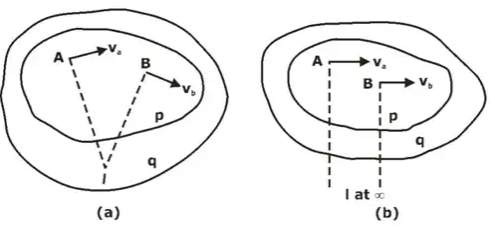Fig.2

If a line is drawn perpendicular to the direction of Va at A, the body can be imagined to rotate about some point on this line. Similarly, the centre of rotation of the body also lies on a line perpendicular to the direction of Vb at B.
If the intersection of the two lines is at I, the body p will be rotating about I at the instant.
This point I is known as the instantaneous centre of velocity or more commonly instantaneous centre of rotation for the body p.
This property is true only for an instant and a new point will become the instantaneous centre at the next instant.
For Fig.14 (b) instantaneous centre will be at infinite at that instant.
Now imagine that the body q is also in motion relative to a third body r. Then the motion of the point I relative to the third body would be the same whether this point is considered on the body p or q. This principle used in Kennedy’s theorem.

1. Notation/Convention
An I-centre is a centre of rotation of a moving body relative to another body. If a body p is in motion relative to a fixed body q, the centre of rotation (I-centre) may be named as pq. However, in case of relative motions, the body q can also be imagined to rotate relative to body p (i.e., as if the body p is fixed) about the same centre. Thus, centre of rotation or I-centre can be named qp also.
This shows that the I-centre of the two body’s p and q in relative motion can be named either pq or qp meaning the same thing.
In general, the I-centre will be named in the ascending order of the alphabets or digits, i.e. 13, 35, pq, qr etc.
2. Number of I-Centres
For two bodies having relative motion between them, there is an I-centre. Thus, in a mechanism, the number of I-centres will be equal to possible pairs of bodies or links.
Let N = Number of I-centres
n = number of bodies or links
Then, N = n(n - 1) / 2
3. Trajectory/Locus of I-Centres while link is in motion
(i) In general, the motion of a link in a mechanism is neither pure rotation nor pure translation. It is a combination of translation and rotation, which we say the link is in general motion.
(ii) Locus of instantaneous centre for a link in its motion is called CENTRODE.
(iii) Locus of instantaneous axis of rotation for a link is called AXODE.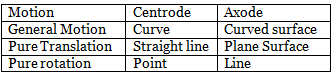Sir Arnhold Kennedy’s Theorem

• Consider three plane bodies p, q and r.
• r being a fixed body, p and q rotate about centre pr and qr respectively relative to the body r. Thus, pr is the I-centre of body p and r whereas qr is the I-centre of body q and r.
• Assume the I-centre of the body p and q at the point pq as shown in Figure below.
• Now, p and q both are moving relative to a third fixed body r. Therefore, the motion of their mutual I-centre pq is to be the same whether this point is considered in the body p or q.
• If the point pq is considered on the body p, its velocity v is perpendicular to the line joining pq and pr. If the point pq is considered on the body q, its velocity vq is perpendicular to the line, joining pq and qr. It is found that the two velocities of the I-centre pq are in different directions which is impossible. Therefore, the I-centre of the bodies p and q cannot be at the assumed position pq.
• The velocities vp and vq of the I-centre will be same only if this centre lies on the line joining pr and qr.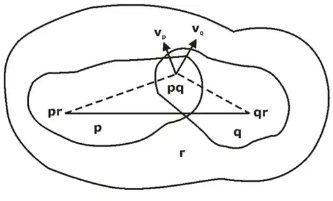Fig.3In other words, if three plane bodies/links have relative motion among themselves, their I-centre must lie on a straight line. This is known as Kennedy’s theorem.

Angular Velocities Ratio Theorem

• When the angular velocity of a link is known and it is required to find the angular velocity of another link, locate their common I-centre. The velocity of this I-centre relative to a fixed third link is the same whether the I-centre is considered on the first or the second link.
• First consider the I-centre to be on the first link and obtain the velocity of the I-centre. Then consider the I-centre to be on the second link and find its angular velocity.
• Let There are two links m and n. To satisfy Kennedy’s Theorem the velocities Vm and Vn of the I-centre will be same.
i.e. Vm = ωm (Imn - I1m) = ωn(Imn - I1n) = Vn
where ωm = angular velocity of link m
ωn = angular velocity of link n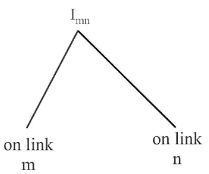Fig. 4

Sign convention: If I1m and I1n lie at the same side of Imn, then direction of rotation of ωm and ωn will be same. If I1m and I1n lie on the opposite side of Imn, then direction of rotation of ωm and ωn will be opposite.

Acceleration

Velocity of a moving body is a vector quantity having magnitude and direction. A change in the velocity requires any of the following conditions to be fulfilled:

• Magnitude change only
• Direction change only
• Both magnitude and direction change

The rate of change of velocity with respect to time is known as acceleration and it acts in the direction of the change in velocity. Thus acceleration is also a vector quantity.

• Let a link AB is rotating about point B with angular velocity ωAB & Angular Acceleration αAB. Now, there will be two components of acceleration of this link AB.
(i) Radial component of acceleration, arAB which will be always directed towards the centre of rotation of the link.
(ii) Tangential component of acceleration, atAB which will act in a direction perpendicular to the link.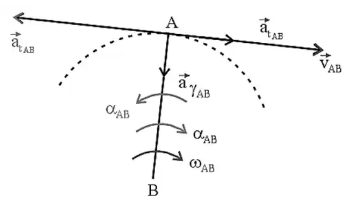Fig.5: Configuration Diagram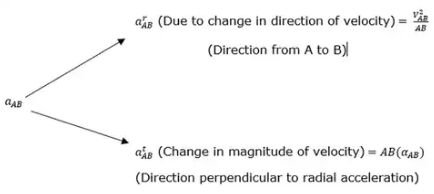Coriolis Acceleration Component

• It is seen that the acceleration of a moving point relative to a fixed body may have two components of acceleration; the centripetal and the tangential. However, in some cases, the point may have its motion relative to a moving body system, for example, motion of a slider on a rotating link.
• The component 2ωv is known as the Coriolis acceleration component.
Magnitude of this acceleration is, ac = 2vω
Where, v = sliding velocity of slider
ω = angular velocity of body on which slider is sliding

To find the direction of Coriolis Acceleration

• Follow below steps to find out the direction of Coriolis acceleration
• Take the sense of direction of ω.
• Rotate the velocity vector in the direction of ω by an angle of 90 degrees if slider is moving outwards.
• Rotate the velocity vector in the direction opposite to that of ω by an angle of 90 degrees if slider is moving inwards.
• Examples: Direction of Coriolis component in various conditions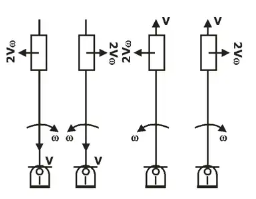Klein’s Construction

• In Klein’s construction, the velocity and the acceleration diagrams are made on the configuration diagram itself.
• The line that represents the crank in the configuration diagram also represents the velocity and the acceleration of its moving end in the velocity and the acceleration diagrams respectively.
• This can only be applied in Basic Single Slider Crank Mechanism when ωcrank is given and αcrank is zero.
Here, OAP = velocity diagram of single slider crank mechanism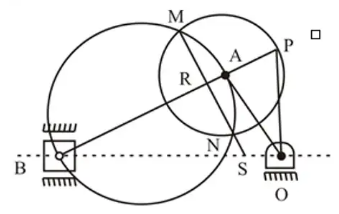Fig.6: Klein’s construction of a single-slider crank mechanism

Both Velocity and acceleration diagram can be summarize by following equations: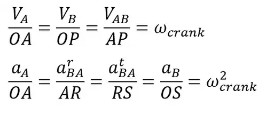Offer running on EduRev: Apply code STAYHOME200 to get INR 200 off on our premium plan EduRev Infinity!

## Theory of Machines (TOM)

94 videos|41 docs|28 tests

,

,

,

,

,

,

,

,

,

,

,

,

,

,

,

,

,

,

,

,

,

,

,

,

;